# TS Polycet (Polytechnic) 2020 Previous Question Paper with Answers And Model Papers With Complete AnalysisTS Polycet (Polytechnic) 2020 Previous Question Paper with Answers And Model Papers With Complete Analysis
TS Polycet (Polytechnic) Previous Year Question Papers And Model Papers:
While preparing for TS Polycet (Polytechnic), candidates must also refer to the previous year question papers of the same. Scoring well in TS Polycet (Polytechnic) and understanding weaknesses and strengths in the respective sections.

TS Polycet (Polytechnic) Previous Year Question Papers can be found on this page in PDF format. Students taking the exam to get into some of the best Polytechnic colleges/institutes in the state of Andhra Pradesh may practice these papers to get a clear idea of the structure of the exam, marking scheme, important topics, etc.

## Section — I MATHEMATICS

Q) 10th term of A.P.: 13, 8, 3, -2,…………… is ___________
A) -32
B) -23
C) 30
D) -30

A) -32
Explanation: 13, 8, 3,-2…….. A.P.
Here a = 13, d = 8 — 13 = —5
Nth term an = a + (n – 1) d
∴ 10th term a10 = 13 + (10 – 1) (-5)
= 13 + 9 (-5)
= 13-45 = -32

Q) If the slope of the line through (2, -7) and (x, 5) is 3, then x =__________
A) 4
B) 5
C) 6
D) 7

C) 6
Explanation: Let A (2, -7), B (x, 5)
Slope of the line passing through
A (x1,y1), B (x2,y2) is
AB=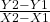Given slope m = 3
3 =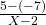3 = 12/x-2
=> 3x-6 = 12
=> 3x = 18
=> x = 6

Q) The centroid of the triangle whose vertices are (3, -5), (-7, 4), (10, -2) is ____________
A) (1, 1)
B) (1,-2)
C) (-2,1)
D) (2,-1)

D) (2,-1)
Explanation: The centroid of the Ale formed by the points A (x1,y1), B (x2,y2), C (x3,y3) is
G = (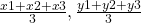)
G = (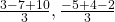)
G = (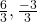)
= (2,-1)

Q) If the roots of 2 x2+ kx + 3 = 0 are real and equal, then k =
A) ±6√2
B) ±4
C) ±2√6
D) ±5

C) ±2√6
Explanation: Given quadratic equation is
2 x2+ kx + 3 = 0
Comparing with
a x2 + bx + c = 0
a = 2, b = k, c = 3
Roots are real and equal, then
b2 – 4ac = 0
=> (k)2 – 4(2)(3) = 0
=> k2 – 24 = 0
k2 = 24
=> k = ± √24 = ±2 √6

Q) Product of the roots of √3 x2 – 2x -√3 =0 is
A) 1
B) -1
C) 2/√3
D) -2/√3

B) -1
Explanation: Given quadratic equation is
√3 x2-2x-√3=0
Comparing with a x2 + bx + c = 0
a = √3, b = —2, c = — √3
Product of the roots = c/a
= -√3/√3
= -1

Q) 4, 7, 10,………….. are in A.P., then stun of 15 terms is _________
A) 385
B) 475
C) 375
D) 325

C) 375
Explanation: 4, 7, 10……….A.P.
Here a = 4, d = 7 -4 = 3, n = 15
Sn = n/2 [2a + (n-1)d] ∴ S15 = 15/2 [2(4) + (15- 1) 3] = 15/2 [8 + 14 x 3] = 15/2 [8 + 42] = 15/2 x 50
= 15 x 25 = 375

Q) The point (2,-3) divides the line segment joining the points (-1, 3), (4; -7) in the ratio_______
A) 3 : 2
B) 2 : 3
C) 8 : 1
D) 1 : 4

A) 3 : 2
Explanation: The ratio in which (x, y) divides the line segment joining (x1,y1), (x2,y2) is
X1 – X : X -X2
(x, y) = (2,-3), (x1,y1) = (-1, 3), (x2,y2) = (4, -7)
= -1 – 2 : 2 – 4
= -3 : -2 = 3 : 2

Q) If (8, 1), (k, —4), (2, -5) are collinear, then k= __________
A) 4
B) 3
C) 2
D) 1

B) 3
Explanation: (8, 1), (k, -4), (2, -5) are collinear, then area of △ABC = 0
X1 (y2-y3)+ X2 (y3-y1)+ X3 (y1-y2) = 0
=> 8(—4 + 5) + k (-5-1) + 2 (1 + 4) = 0
=> 8(1) + k(-6) +2 (5) = 0
=> 8-6k+10 = 0
=>18-6k = 0
=> -6k = -18
=>k = 3

Q) 8 x2-6x-9 = ________
A) (2x-3)(x-3)
B) (2x-3)(x + 1)
C) (2x+1) (x- 1)
D) (2x – 3) (4x + 3)

D) (2x – 3) (4x + 3)
Explanation: 8 x2-6x-9
= 8 x2– 12x + 6x-9
= 4x(2x-3) + 3 (2x-3)
= (2x – 3) (4x + 3)

Q) 1,-1/2,1/4,…………………. are in G.P., then find 8th term.
A) 1/128
B) 1/64
C) -1/128
D) -1/64